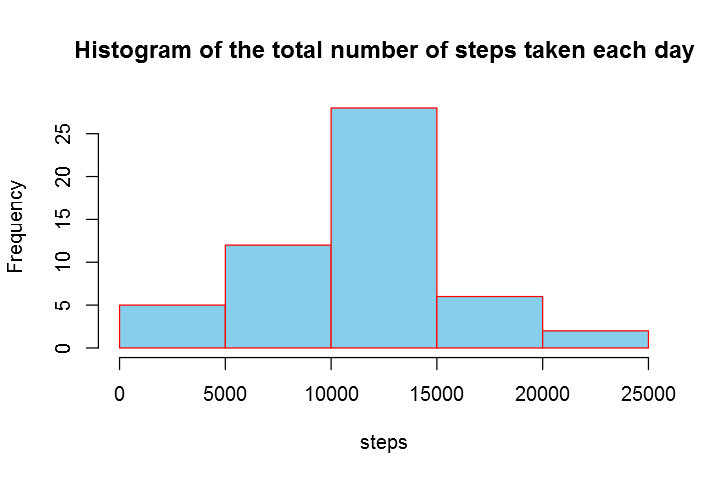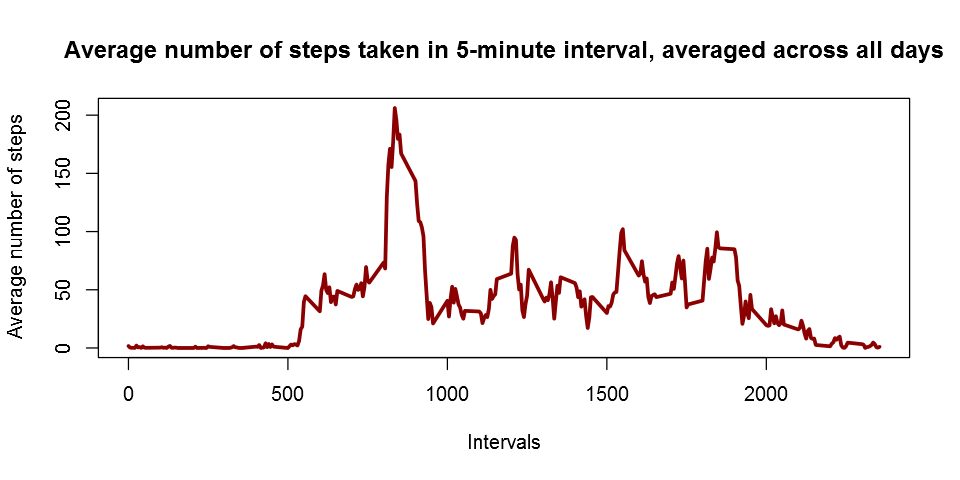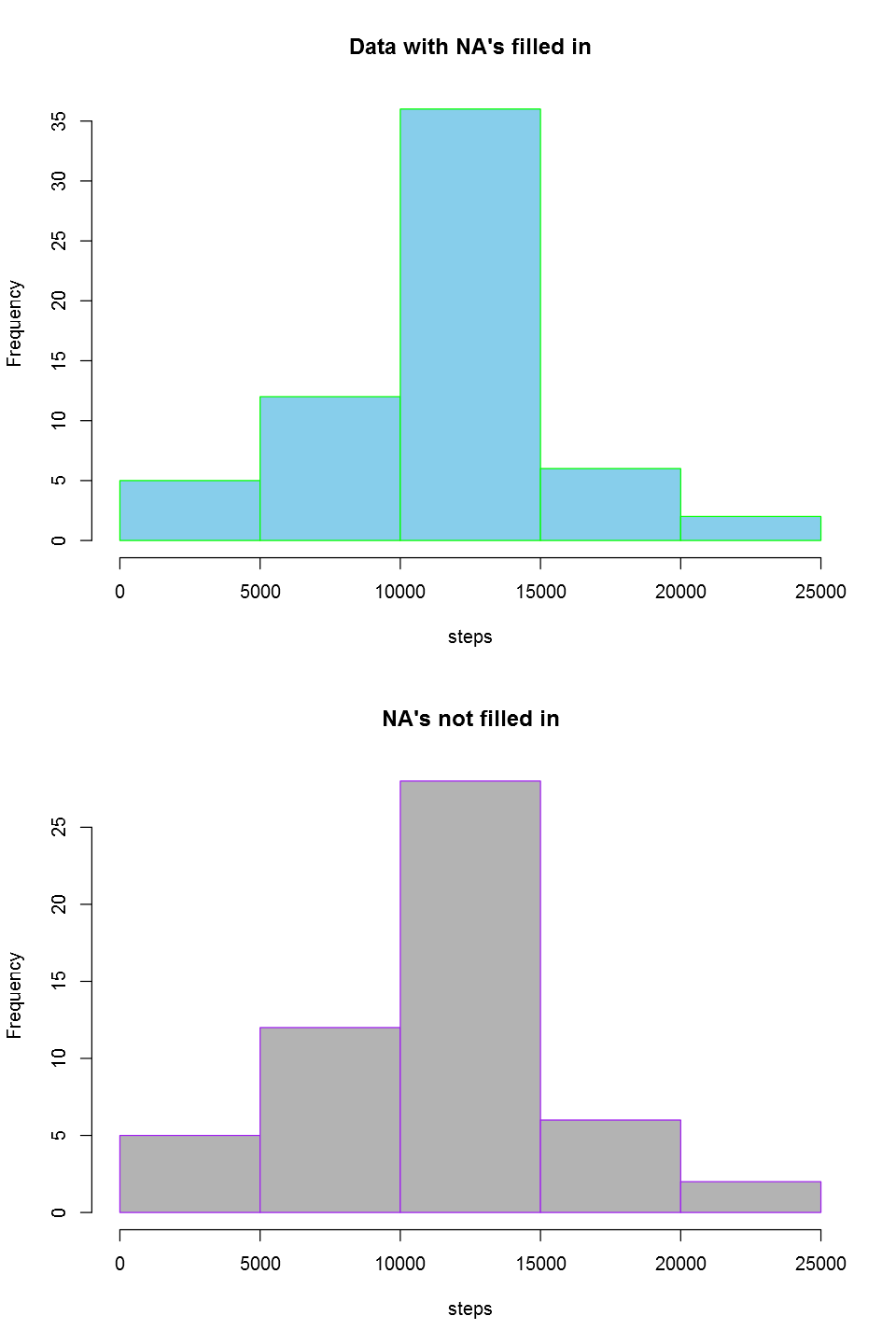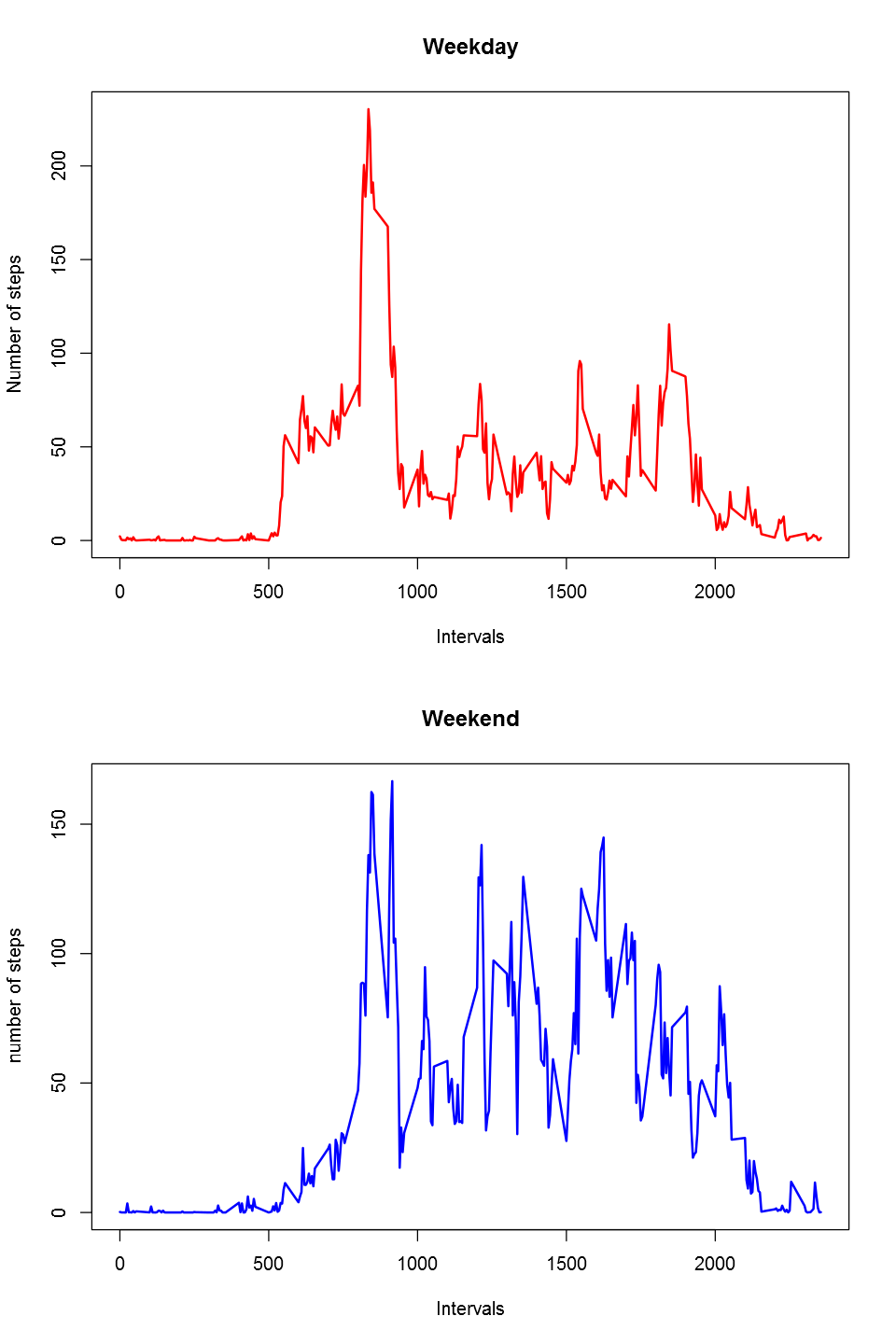# Fisseha Berhane, PhD

#### Data Scientist443-970-2353[email protected] CV Resume### Reproducible Research with R¶

It is now possible to collect a large amount of data about personal movement using activity monitoring devices such as a Fitbit (http://www.fitbit.com), Nike Fuelband (http://www.nike.com/us/en_us/c/nikeplusfuelband), or Jawbone Up (https://jawbone.com/up). These type of devices are part of the “quantified self” movement – a group of enthusiasts who take measurements about themselves regularly to improve their health, to find patterns in their behavior, or because they are tech geeks. But these data remain underutilized both because the raw data are hard to obtain and there is a lack of statistical methods and software for processing and interpreting the data. This assignment makes use of data from a personal activity monitoring device. This device collects data at 5 minute intervals through out the day. The data consists of two months of data from an anonymous individual collected during the months of October and November, 2012 and include the number of steps taken in 5 minute intervals each day.

First, let’s clear the workspace and load a library that helps to add hour and minute

In :
rm(list=ls())    # clear workspace

library(dplyr)


Then, let’s load the data and understand its contents

In :
data<-read.csv("activity.csv", sep=',', header=TRUE)  # loading data

# Let us have a look at the data dimensions, variables

names(data)

Out:
1. "steps"
2. "date"
3. "interval"
In :
dim(data)

Out:
1. 17568
2. 3
In :
str(data)

'data.frame':	17568 obs. of  3 variables:
$steps : int NA NA NA NA NA NA NA NA NA NA ...$ date    : Factor w/ 61 levels "2012-10-01","2012-10-02",..: 1 1 1 1 1 1 1 1 1 1 ...
$interval: int 0 5 10 15 20 25 30 35 40 45 ...  In : head(data)  Out: stepsdateinterval 1NA2012-10-010 2NA2012-10-015 3NA2012-10-0110 4NA2012-10-0115 5NA2012-10-0120 6NA2012-10-0125 In : tail(data)  Out: stepsdateinterval 17563NA2012-11-302330 17564NA2012-11-302335 17565NA2012-11-302340 17566NA2012-11-302345 17567NA2012-11-302350 17568NA2012-11-302355 #### Process/transform the data (if necessary) into a format suitable for your analysis¶ In : # Let's add hour and minute data <- mutate(data, hour = interval %/% 100, minute = interval %% 100)  #### What is mean total number of steps taken per day?¶ For this part of the assignment, missing values will be ignored! Calculate the total number of steps taken per day In : daily<-c() # This will be the total number of steps taken per day for (i in 1:61){ # total number of days in October and November is 31+30=61 start<-(i-1)*288+1 # 288 five-minute steps in a day; 24*60/5=288 last<-(i-1)*288+288 temp<-data[start:last,1] # extracting all 5-minute steps for each day daily<-c(daily,sum(temp)) # concatenating the daily totals }  Make a histogram of the total number of steps taken each day In : daily_noNA<-daily[!is.na(daily)] # 8 NA's are removed hist(daily_noNA, xlab="steps",ylab="Frequency",col="skyblue",border="red", main="Histogram of the total number of steps taken each day")Calculate and report the mean and median of the total number of steps taken per day The mean of total number of steps taken per day is: In : mean(daily,na.rm=T)  Out: 10766.1886792453 The median of total number of steps taken per day is: In : median(daily,na.rm=T)  Out: 10765 ##### What is the average daily activity pattern?¶ Make a time series plot (i.e. type = “l”) of the 5-minute interval (x-axis) and the average number of steps taken, averaged across all days (y-axis) In : x<-data[,1] # number of steps in 5-minute intevals y<-matrix(x,288,61) # so as to get average of 5-minute intevals across all days five_average<-apply(y,1,mean,na.rm=TRUE) # 5-minute interval average number of steps taken, # averaged across all days plot(data$interval[1:288],five_average, type='l',col='darkred',
xlab='Intervals',lwd=3,
ylab='Average number of steps',
main ='Average number of steps taken in 5-minute interval, averaged across all days')Which 5-minute interval, on average across all the days in the dataset, contains the maximum number of steps?

In :
hr<-data$hour[1:288] min<-data$minute[1:288]

hr_max<-hr[which(five_average==max(five_average))]
min_max<-min[which(five_average==max(five_average))]

cat('The maximum number of steps occurs at',hr_max,':',min_max,'AM')

The maximum number of steps occurs at 8 : 35 AM

#### Imputing missing values¶

Calculate and report the total number of missing values in the dataset (i.e. the total number of rows with NAs)

The total number of missing values is:

In :
sum(is.na(data[,1]))

Out:
2304

Devise a strategy for filling in all of the missing values in the dataset. The strategy does not need to be sophisticated. For example, you could use the mean/median for that day, or the mean for that 5-minute interval, etc.

I will fill in missing values using the mean of the 5-minute interval

In :
# five_average is the 5-minute average across all days as shown in plotting the histogram above

# Then we can fill in all mising values with the average for that 5-minute interval across all days

# Let us replicate the 5-minute interval average over the number of days

five_average_rep<- rep(five_average,61)

data1<-data   # creating a copy of the datset so as to not mess up the original data

for (i in 1:length(data1[,1])){  # there are 61 days

if(is.na(data1[i,1])==TRUE){
data1[i,1]= five_average_rep[i]  # missing values replaced
}}


Create a new dataset that is equal to the original dataset but with the missing data filled in.

data1 in question 2 above is the same as the original dataset, with all missing values filled in by the mean for that 5-minute interval across all days.

Make a histogram of the total number of steps taken each day and Calculate and report the mean and median total number of steps taken per day. Do these values differ from the estimates from the first part of the assignment? What is the impact of imputing missing data on the estimates of the total daily number of steps?

In :
# Calculate the total number of steps taken per day using the data with filled NA's

daily1<-c()

for (i in 1:61){              #  the total number of days in October and November is 31+30=61
start<-(i-1)*288+1        #  there are 288 five-minute steps in a day; 24*60/5=288
last<-(i-1)*288+288
temp<-data1[start:last,1]    # extracting all 5-minute steps for each day
daily1<-c(daily1,sum(temp))   # concatenating the daily totals
}


Histograms of the total number of steps taken each day using both the original data and missing data replaced with 5-minute average across all days

In :
par(mfrow=c(2,1))

hist(daily1, xlab="steps",ylab="Frequency",
main="Data with NA's filled in",border='green',col="skyblue")

hist(daily_noNA, xlab="steps",ylab="Frequency",
main="NA's not filled in",border='purple',col="gray70",)In :
# The mean of  total number of steps taken per day is:

mean(daily1)

Out:
10766.1886792453
In :
# The median of  total number of steps taken per day is:

median(daily1)

Out:
10766.1886792453

Do these values differ from the estimates from the first part of the assignment? What is the impact of imputing missing data on the estimates of the total daily number of steps?

Yes, they show diferreneces in the median and in the histograms. imputing missing data on the estimates of the total daily number of steps changes the median, and the distribution as as can be seen from the histograms.Based on the method used for filling in missing values, we can get different mean and median values. The histogram can also be different based on the strategy we used to fill in the missing values

#### Are there differences in activity patterns between weekdays and weekends?¶

Create a new factor variable in the dataset with two levels - “weekday” and “weekend” indicating whether a given date is a weekday or weekend day.

In :
data1$date<-as.Date(data1$date)

data1$day<-weekdays(data1$date)

data1_weekdays<-data1[(!data1$day %in% c("Saturday","Sunday")),] # weekdays data1_weekend<-data1[(data1$day %in% c("Saturday","Sunday")),]   #  weekend

weekday_steps<-data1_weekdays[,1]

temp<-matrix(weekday_steps,nrow=288)

weekday_steps_average<-apply(temp,1,mean)

weekend_steps<-data1_weekend[,1]

temp<-matrix(weekend_steps,nrow=288)

weekend_steps_average<-apply(temp,1,mean)


Make a panel plot containing a time series plot (i.e. type = “l”) of the 5-minute interval (x-axis) and the average number of steps taken, averaged across all weekday days or weekend days (y-axis)

In :
par(mfrow=c(2,1))

plot(data$interval[1:288],weekday_steps_average, type="l",xlab='Intervals',ylab="Number of steps", col='red',lwd=2, main="Weekday") plot(data$interval[1:288],weekend_steps_average, type="l", xlab='Intervals',ylab="number of steps",
col='blue',lwd=2,main="Weekend")